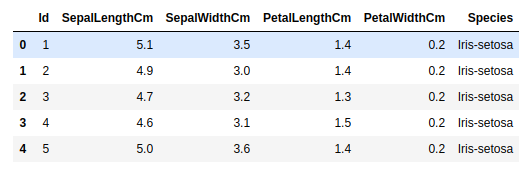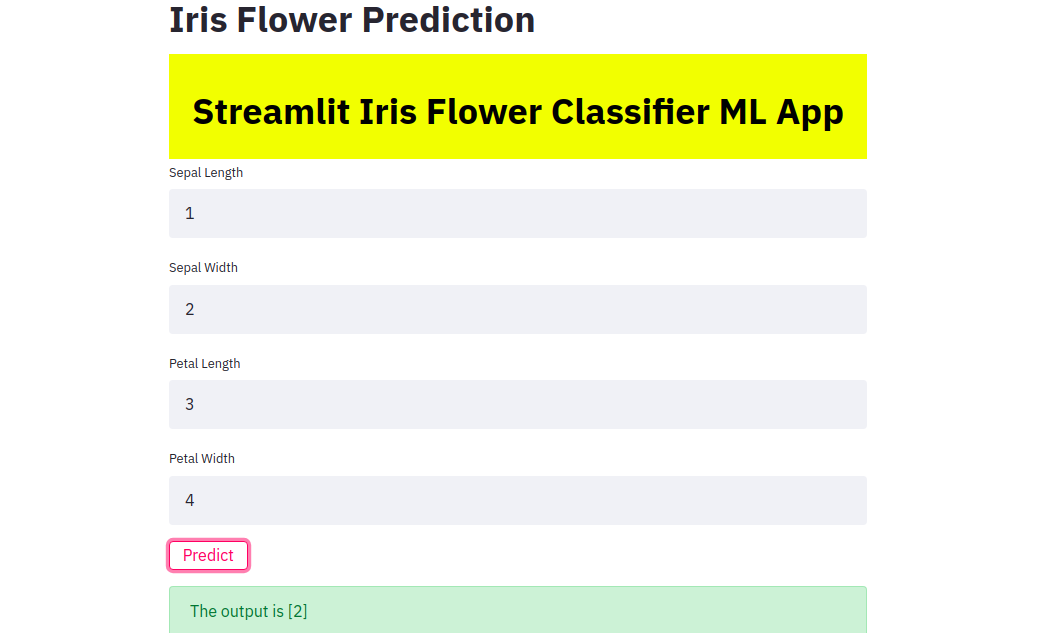Related Articles
Deploy a Machine Learning Model using Streamlit Library
• Last Updated : 02 Sep, 2020

Machine Learning:
A computer is able to learn from experience without being explicitly programmed. Machine Learning is one of the top fields to enter currently and top companies all over the world are using it for improving their services and products. But there is no use of a Machine Learning model which is trained in your Jupyter Notebook. And so we need to deploy these models so that everyone can use them. In this article, we will first train an Iris Species classifier and then deploy the model using Streamlit which is an open-source app framework used to deploy ML models easily.

Streamlit Library:
Streamlit lets you create apps for your machine learning project using simple python scripts. It also supports hot-reloading, so that your app can update live as you edit and save your file. An app can be built in a few lines of code only(as we will see below) using the Streamlit API. Adding a widget is the same as declaring a variable. There is no need to write a backend, define different routes or handle HTTP requests. It is easy to deploy and manage. More information can be found on their website – https://www.streamlit.io/

So first we will train our model. We will not do much preprocessing as the main aim of this article is not to make an accurate ML model but to show its deployment.

Firstly we need to install the following –

pip install pandas
pip install numpy
pip install sklearn
pip install streamlit

The dataset can be found here: https://www.kaggle.com/uciml/iris

Code:

 `import` `pandas as pd ` `import` `numpy as np ` ` `  `df ``=` `pd.read_csv(``'BankNote_Authentication.csv'``) ` `df.head() `

Output:Now we drop the Id column first as it is not important for classifying the Iris species. Then we will split the dataset into training and testing dataset and will use a Random Forest Classifier. You can use any other classifier of your choice, for example, logistic regression, support vector machine, etc.

Code:

 `# Dropping the Id column ` `df.drop(``'Id'``, axis ``=` `1``, inplace ``=` `True``) ` ` `  `# Renaming the target column into numbers to aid training of the model ` `df[``'Species'``]``=` `df[``'Species'``].``map``({``'Iris-setosa'``:``0``, ``'Iris-versicolor'``:``1``, ``'Iris-virginica'``:``2``}) ` ` `  `# splitting the data into the columns which need to be trained(X) and the target column(y) ` `X ``=` `df.iloc[:, :``-``1``] ` `y ``=` `df.iloc[:, ``-``1``] ` ` `  `# splitting data into training and testing data with 30 % of data as testing data respectively ` `from` `sklearn.model_selection ``import` `train_test_split ` `X_train, X_test, y_train, y_test ``=` `train_test_split(X, y, test_size ``=` `0.3``, random_state ``=` `0``) ` ` `  `# importing the random forest classifier model and training it on the dataset ` `from` `sklearn.ensemble ``import` `RandomForestClassifier ` `classifier ``=` `RandomForestClassifier() ` `classifier.fit(X_train, y_train) ` ` `  `# predicting on the test dataset ` `y_pred ``=` `classifier.predict(X_test) ` ` `  `# finding out the accuracy ` `from` `sklearn.metrics ``import` `accuracy_score ` `score ``=` `accuracy_score(y_test, y_pred) `

We get an accuracy of 95.55% which is pretty good.

Now, in order to use this model to predict other unknown data, we need to save it. We can save it by using pickle, which is used for serializing and deserializing a Python object structure.

Code:

 `# pickling the model ` `import` `pickle ` `pickle_out ``=` `open``(``"classifier.pkl"``, ``"wb"``) ` `pickle.dump(classifier, pickle_out) ` `pickle_out.close()`

There will be a new file created called “classifier.pkl” in the same directory. Now we can get down to using Streamlit to deploy the model –

Paste the below code into another python file.
Code:

 `import` `pandas as pd ` `import` `numpy as np ` `import` `pickle ` `import` `streamlit as st ` `from` `PIL ``import` `Image ` ` `  `# loading in the model to predict on the data ` `pickle_in ``=` `open``(``'classifier.pkl'``, ``'rb'``) ` `classifier ``=` `pickle.load(pickle_in) ` ` `  `def` `welcome(): ` `    ``return` `'welcome all'` ` `  `# defining the function which will make the prediction using  ` `# the data which the user inputs ` `def` `prediction(sepal_length, sepal_width, petal_length, petal_width):   ` `  `  `    ``prediction ``=` `classifier.predict( ` `        ``[[sepal_length, sepal_width, petal_length, petal_width]]) ` `    ``print``(prediction) ` `    ``return` `prediction ` `     `  ` `  `# this is the main function in which we define our webpage  ` `def` `main(): ` `      ``# giving the webpage a title ` `    ``st.title(``"Iris Flower Prediction"``) ` `     `  `    ``# here we define some of the front end elements of the web page like  ` `    ``# the font and background color, the padding and the text to be displayed ` `    ``html_temp ``=` `""" ` `    ``
` `    ``

Streamlit Iris Flower Classifier ML App

` `    ``
` `    ``"""` `     `  `    ``# this line allows us to display the front end aspects we have  ` `    ``# defined in the above code ` `    ``st.markdown(html_temp, unsafe_allow_html ``=` `True``) ` `     `  `    ``# the following lines create text boxes in which the user can enter  ` `    ``# the data required to make the prediction ` `    ``sepal_length ``=` `st.text_input(``"Sepal Length"``, ``"Type Here"``) ` `    ``sepal_width ``=` `st.text_input(``"Sepal Width"``, ``"Type Here"``) ` `    ``petal_length ``=` `st.text_input(``"Petal Length"``, ``"Type Here"``) ` `    ``petal_width ``=` `st.text_input(``"Petal Width"``, ``"Type Here"``) ` `    ``result ``=``"" ` `     `  `    ``# the below line ensures that when the button called 'Predict' is clicked,  ` `    ``# the prediction function defined above is called to make the prediction  ` `    ``# and store it in the variable result ` `    ``if` `st.button(``"Predict"``): ` `        ``result ``=` `prediction(sepal_length, sepal_width, petal_length, petal_width) ` `    ``st.success(``'The output is {}'``.``format``(result)) ` `    `  `if` `__name__``=``=``'__main__'``: ` `    ``main() `

You can run this by typing the following command in the terminal –

streamlit run app.pyapp.py is the name of the file where we wrote the Streamlit code.

The website will open in your browser and then you can test it. This method can be used to deploy other machine and deep learning models too.My Personal Notes arrow_drop_up
Recommended Articles
Page :